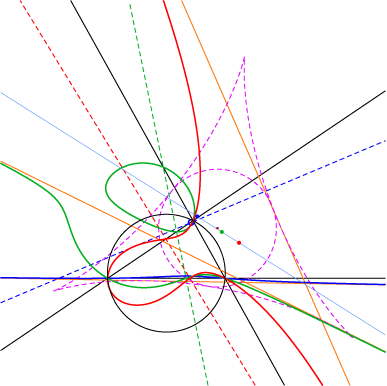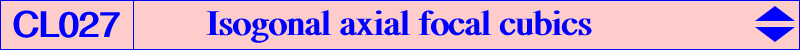All isogonal circular nK are focal cubics with singular focus F on the circumcircle (see Special Isocubics § 4.1.2). They form a net of cubics. For a given focus F on the circumcircle, these cubics form a pencil of focal cubics containing one central cubic and one axial cubic i.e. a cubic invariant under an axial symmetry with axis L. The real asymptote must be parallel to L and the real point at infinity of the cubic must be a flex. Obviously, F lies on L. The pencil also contains four strophoids with node one in/excenter. See a generalization in the page P-conical cubics.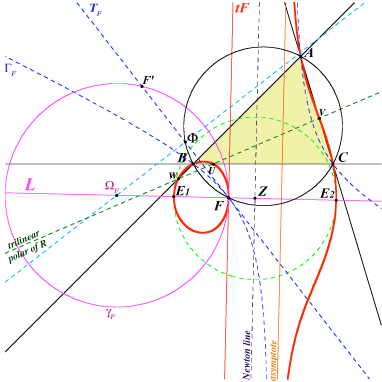The perpendicular at F to the Simson line of F is the tangent tF at F to the axial cubic. L is the parallel at F to this Simson line. The isogonal conjugate of tF is the circum-conic Gamma_F passing through F and having one asymptote parallel to tF. This asymptote is the real asymptote of the cubic. The median line of this asymptote and tF is the Newton line of a quadrilateral such that the locus of foci of inscribed conics in this quadrilateral is the axial cubic. These conics are obviously centered on this Newton line. Recall that CL001 is the class of central isogonal focal cubics obtained when the inconics are centered on tF and that CL003 is the class of isogonal strophoids obtained when the inconics are centered on a parallel to tF at one in/excenter. See a figure with F = X(99) below.The homothety h(A,2) transforms this Newton line into another line meeting BC at U. Define V and W similarly. The line UVW is the trilinear polar of the root R of the cubic. The tangent T_F at F to Gamma_F meets the circumcircle again at Phi and the perpendicular at Phi to T_F meets L at Omega_F, center of the circle gamma_F which is the polar conic of F in the cubic. The Newton line meets L at Z. The circle C_Z centered at Z and orthogonal to gamma_F meets L at E1, E2 centers of anallagmaty of the cubic. These are two isogonal conjugate points therefore also lying to the circum-conic isogonal transform of L. This conic contains the antipode of F on the circumcircle and the infinite point of tF. Naturally, E1 and E2 also lie on pK(X6, F). See table 17 for such cubics. In order to realize the construction of the cubic, draw a circle centered on L and orthogonal to C_Z then the tangents from F to this circle. The contacts are two points M, N on the cubic symmetric in L. Their isogonal conjugates M*, N* are also symmetric in L and the midpoints of MM*, NN*, MN*, NM* lie on the Newton line. The lines MN* and NM* contain F hence M, M* and N, N* are also conjugated points on the cubic i.e. the bisectors of FM, FM* and FN, FN* are L and tF i.e. M, M* and N, N* share the same tangentials tanM and tanN respectively. Let M1, M2 be the inverses of M in the inversions with poles E1, E2 swapping F and E2, F and E1 respectively. M1 and M2 are isogonal conjugate points on the cubic. They are also the inverses of M* in the same inversions with poles E2, E1 respectively. The points N1, N2 are defined similarly. The points M3 = MM*/\N1N2, M4 = MN1/\M*N2, M4* = MN2/\M*N1 are three other points on the cubic and M, M*, N1, N2 share the same tangential tanM which is the isogonal conjugate of M3. Hence, it is easy to obtain the tangents at M, M*, N1, N2 to the cubic and, by symmetry, we also know the tangents at N, N*, M1, M2. This shows that the cubic is a pK with pivot tanM with respect to the triangle M3M4M4* invariant in the isoconjugation with fixed points M, M*, N1, N2.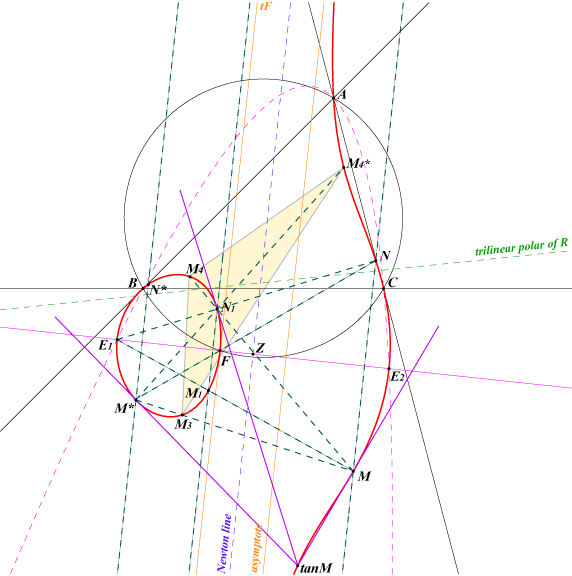The Steiner family of isogonal focal nKs The following figure shows several members of the pencil of isogonal focal nKs with singular focus X(99). All these cubics have their root on the line X(2)X(39) and meet their real asymptote on the hyperbola (H) which is the isogonal transform of the line X(99)X(512). The cubic with root X(2) is degenerate into the circumcircle and the line at infinity. With a root X(3291), the cubic is a nK0. Each cubic has two centers of anallagmaty lying on K035 = pK(X6, X99), the Steiner cubic (see also table 17). Indeed, these centers must lie : on the bisectors of the line X(99)X(512) (the real asymptote of K084) and the tangent at F to the focal cubic, on the circum-conics which are their isogonal conjugates.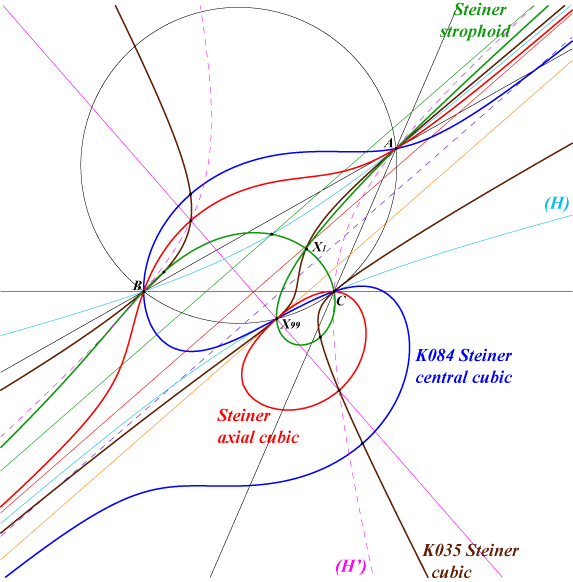Isogonal axial nK0s When the root R lies on the orthic axis, the cubic is a nK0. There are six (not always real) roots Ri for which the cubic is an axial cubic. The corresponding infinite points (which are flexes on the cubics) are those of the second tangents (apart the orthic axis) drawn through Ri to the parabola (P) and obviously, the singular foci Fi are their isogonal conjugates. (P) is the parabola with focus X(111) and directrix the line X(1499)X(1513). (P) is tangent to the orthic axis at X(230). The real asymptotes are tangent to the deltoid with center X(1351), the reflection of O about K, which is the image in this same reflection of the deltoid envelope of the axes of inscribed parabolas.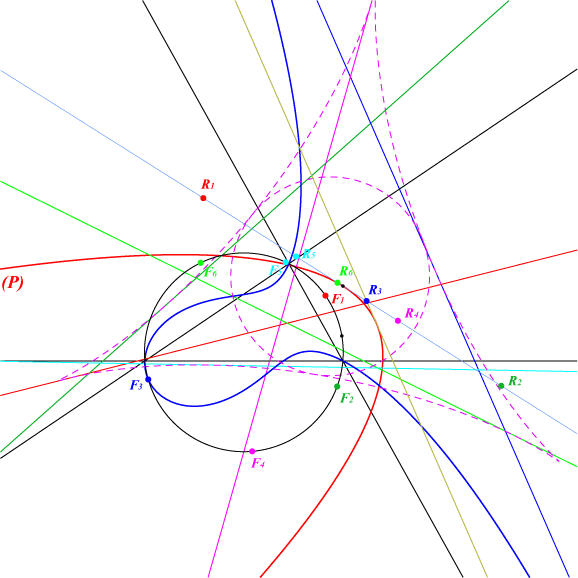A configuration with six real axial nK0s in two separate figures.